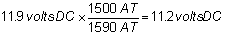MAGNETIC SENSOR SYSTEMS

How To Use This Catalog

 For each solenoid, a coil data table, and a set of force versus stroke curves are presented.  By knowing the size, stroke and required force, a number for ampere turns can be obtained from the curves, which in turn, by reference to coil data, points out the amount of power consumption. By choosing the proper input voltage to the solenoid from the related column, the wire size will be obtained, which defines the type of solenoid to be used. Example: In an electro-mechanical system, space allows for a 1.00 inch diameter by 2.00 inch long solenoid, which must exert 50.0 ounces of force at a stroke of 0.200 inches. The solenoid will be used intermittently and the input voltage is approximately 12.0 volts DC. The basic number for this solenoid is S-20-100. Force versus stroke curves show that approximately 1500 ampere turns is required in order to apply 50 ounces at 0.200 inches. The closest ampere turns recorded in the chart is 1590AT. A simple interpolation will show the proper voltage for the solenoid.The wire gauge specified in the chart corresponding to 11.9  volts is 26 awg.  In this example no safety factor was considered. Considering a 30% safety factor is a good engineering practice. The force will be dropped slightly as the solenoid gets warmer (see Selection Factors, Temperature). It should also be noted that the force versus stroke curves are typical, for nominal conditions.   How To Order The part number for the solenoid in the above example is: